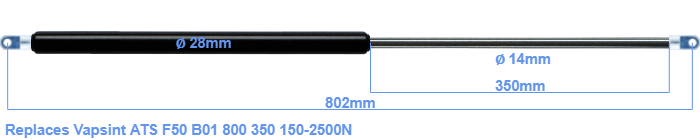# Replacement for Vapsint ATS F50 B01 800 350 150-2500N

\$56.36

Replacement gas spring for the Vapsint ATS F50 B01 800 350 150-2500 Newton. The eye has a thickness of 10mm and a hole diameter of 8.1mm. Brand: Hahn.
 Force Choose an option150 Newton200 Newton250 Newton300 Newton350 Newton400 Newton450 Newton500 Newton550 Newton600 Newton650 Newton700 Newton750 Newton800 Newton850 Newton900 Newton950 Newton1000 Newton1050 Newton1100 Newton1150 Newton1200 Newton1250 Newton1300 Newton1350 Newton1400 Newton1450 Newton1500 Newton1550 Newton1600 Newton1650 Newton1700 Newton1750 Newton1800 Newton1850 Newton1900 Newton1950 Newton2000 Newton2050 Newton2100 Newton2150 Newton2200 Newton2250 Newton2300 Newton2350 Newton2400 Newton2450 Newton2500 NewtonClear
This gas spring is also known as Brimotech 10520006.The bore of the house of this replacement gas spring is 28 mm. The cross section of the rod is 14 mm. The stroke (the retracting part) has a length of 350 mm. In total, the length is 802 millimeter. This is the distance between the centers of the mounting parts. Without the mounting parts this gas spring is 748 mm long (thread to thread). This gas spring (ATS F50 B01 800 350) has a force of 150-2500 Newton. Attention: this is not an official Vapsint gas spring, but a replacement gas spring. This a gas spring with the brand Stabilus Industry Line. Nevertheless, the force and dimensions equal.